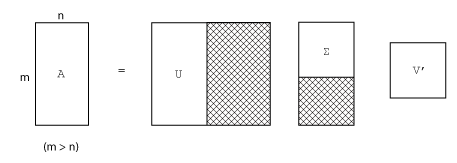## Singular Values

A singular value and corresponding singular vectors of a rectangular matrix A are, respectively, a scalar σ and a pair of vectors u and v that satisfy

`$\begin{array}{l}Av=\sigma u\\ {A}^{H}u=\sigma v,\end{array}$`

where ${A}^{H}$ is the Hermitian transpose of A. The singular vectors u and v are typically scaled to have a norm of 1. Also, if u and v are singular vectors of A, then -u and -v are singular vectors of `A` as well.

The singular values σ are always real and nonnegative, even if A is complex. With the singular values in a diagonal matrix Σ and the corresponding singular vectors forming the columns of two orthogonal matrices U and V, you obtain the equations

`$\begin{array}{l}AV=U\Sigma \\ {A}^{H}U=V\Sigma .\end{array}$`

Since U and V are unitary matrices, multiplying the first equation by ${V}^{H}$ on the right yields the singular value decomposition equation

`$A=U\Sigma {V}^{H}.$`

The full singular value decomposition of an m-by-n matrix involves:

• m-by-m matrix U

• m-by-n matrix Σ

• n-by-n matrix V

In other words, U and V are both square, and Σ is the same size as A. If A has many more rows than columns (`m > n`), then the resulting `m`-by-`m` matrix U is large. However, most of the columns in U are multiplied by zeros in Σ. In this situation, the economy-sized decomposition saves both time and storage by producing an m-by-n U, an n-by-n Σ and the same V:The eigenvalue decomposition is the appropriate tool for analyzing a matrix when it represents a mapping from a vector space into itself, as it does for an ordinary differential equation. However, the singular value decomposition is the appropriate tool for analyzing a mapping from one vector space into another vector space, possibly with a different dimension. Most systems of simultaneous linear equations fall into this second category.

If A is square, symmetric, and positive definite, then its eigenvalue and singular value decompositions are the same. But, as A departs from symmetry and positive definiteness, the difference between the two decompositions increases. In particular, the singular value decomposition of a real matrix is always real, but the eigenvalue decomposition of a real, nonsymmetric matrix might be complex.

For the example matrix

```A = [9 4 6 8 2 7];```

the full singular value decomposition is

```[U,S,V] = svd(A) U = -0.6105 0.7174 0.3355 -0.6646 -0.2336 -0.7098 -0.4308 -0.6563 0.6194 S = 14.9359 0 0 5.1883 0 0 V = -0.6925 0.7214 -0.7214 -0.6925```

You can verify that `U*S*V'` is equal to `A` to within round-off error. For this small problem, the economy size decomposition is only slightly smaller.

```[U,S,V] = svd(A,"econ") U = -0.6105 0.7174 -0.6646 -0.2336 -0.4308 -0.6563 S = 14.9359 0 0 5.1883 V = -0.6925 0.7214 -0.7214 -0.6925```

Again, `U*S*V'` is equal to `A` to within round-off error.

### Batched SVD Calculation

If you need to decompose a large collection of matrices that have the same size, it is inefficient to perform all of the decompositions in a loop with `svd`. Instead, you can concatenate all of the matrices into a multidimensional array and use `pagesvd` to perform singular value decompositions on all of the array pages with a single function call.

FunctionUsage
`pagesvd`Use `pagesvd` to perform singular value decompositions on the pages of a multidimensional array. This is an efficient way to perform SVD on a large collection of matrices that all have the same size.

For example, consider a collection of three 2-by-2 matrices. Concatenate the matrices into a 2-by-2-by-3 array with the `cat` function.

```A = [0 -1; 1 0]; B = [-1 0; 0 -1]; C = [0 1; -1 0]; X = cat(3,A,B,C);```

Now, use `pagesvd` to simultaneously perform the three decompositions.

`[U,S,V] = pagesvd(X);`

For each page of `X`, there are corresponding pages in the outputs `U`, `S`, and `V`. For example, the matrix `A` is on the first page of `X`, and its decomposition is given by `U(:,:,1)*S(:,:,1)*V(:,:,1)'`.

### Low-Rank SVD Approximations

For large sparse matrices, using `svd` to calculate all of the singular values and singular vectors is not always practical. For example, if you need to know just a few of the largest singular values, then calculating all of the singular values of a 5000-by-5000 sparse matrix is extra work.

In cases where only a subset of the singular values and singular vectors are required, the `svds` and `svdsketch` functions are preferred over `svd`.

FunctionUsage
`svds`Use `svds` to calculate a rank-k approximation of the SVD. You can specify whether the subset of singular values should be the largest, the smallest, or the closest to a specific number. `svds` generally calculates the best possible rank-k approximation.
`svdsketch`Use `svdsketch` to calculate a partial SVD of the input matrix satisfying a specified tolerance. While `svds` requires that you specify the rank, `svdsketch` adaptively determines the rank of the matrix sketch based on the specified tolerance. The rank-k approximation that `svdsketch` ultimately uses satisfies the tolerance, but unlike `svds`, it is not guaranteed to be the best one possible.

For example, consider a 1000-by-1000 random sparse matrix with a density of about 30%.

```n = 1000; A = sprand(n,n,0.3);```

The six largest singular values are

```S = svds(A) S = 130.2184 16.4358 16.4119 16.3688 16.3242 16.2838```

Also, the six smallest singular values are

```S = svds(A,6,"smallest") S = 0.0740 0.0574 0.0388 0.0282 0.0131 0.0066```

For smaller matrices that can fit in memory as a full matrix, `full(A)`, using `svd(full(A))` might still be quicker than `svds` or `svdsketch`. However, for truly large and sparse matrices, using `svds` or `svdsketch` becomes necessary.